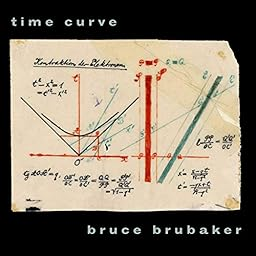album

# Time Curve: Music for Piano by Philip Glass and William Duckworth

18 songs (1 hour and 10 minutes) Released on June 16, 2009
 1 Six Etudes for Piano - q = 168 4:16 2 Six Etudes for Piano - q = 108 6:51 3 Six Etudes for Piano - q = 132 4:27 4 Six Etudes for Piano - q = 104 3:48 5 Six Etudes for Piano - q = 96 4:48 6 Six Etudes for Piano - q = 176 6:51 7 The Time Curve Preludes, Book I - q = 104 3:17 8 The Time Curve Preludes, Book I: II - q = 104 2:04 9 The Time Curve Preludes, Book I: III - q = 126 1:54 10 The Time Curve Preludes, Book I: IV - q = 132 2:19 11 The Time Curve Preludes, Book I - q = 132 2:24 12 The Time Curve Preludes, Book I: VI - q = 102 5:12 13 The Time Curve Preludes, Book I: VII - q = 72 2:43 14 The Time Curve Preludes, Book I: VIII - q = 76 2:49 15 The Time Curve Preludes, Book I: IX - q = 96 2:51 16 The Time Curve Preludes, Book I - q = 192 2:25 17 The Time Curve Preludes, Book I: XI - q = 80 - Freely, with great rubato 6:51 18 The Time Curve Preludes, Book I: XII - q = 124 4:54